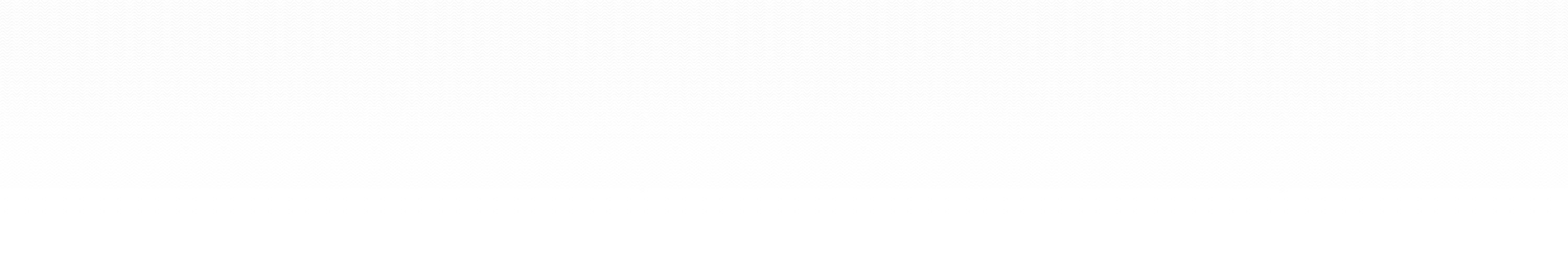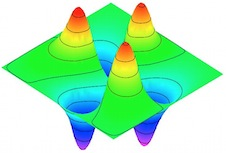### MATH 4503/6503 general informationThe principal goal of this course is to give students “hands-on” experience in solving ordinary and partial differential equations (ODEs and PDEs) using finite difference methods. We will also discuss finite element methods (time permitting). The emphasis will be on practical scientific computing; i.e., the construction of numerical algorithms to deal with actual problems in the physical, biological or engineering sciences using software such as Maple.A 2D finite element simulation of a vibrating drum with a hole cut out